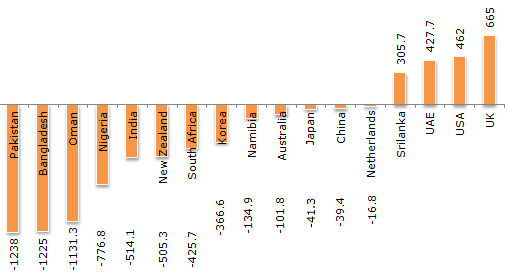# Data Interpretation - Bar Charts

Directions to Solve

The total of the first three deficit countries (in Rs. crores) = 3594.3. The total of next five deficit countries (in Rs. crores) = 2588.5. The total of the last five deficit countries (in Rs. crores) = 334.2.

Cumulative Trade Deficit/Surplus of Countries for the Year 2006 - 2007 (All figures in Rs. Crores).1.
The ratio between the difference between the highest and the lowest of the surplus countries to the difference between Bangladesh and Oman is ?
3.44
2.96
4.5
3.83
Explanation:
The required answer will be equal to 360/94 = 3.829.

2.
The average of the total deficit of the middle five deficit is closest to the deficit of which country ?
Korea
South Africa
UAE
None of these
Explanation:

The middle five deficit countries are India, New Zealand, South Africa, Korea and Namibia.

Their average deficit is (1946.6/5) = 389.32. Korea's deficit is close to this value.

3.
The state whose surplus is nearly equal to the average of the four surplus countries is
Sri Lanka
UAE
USA
UK
Explanation:
The average trade surplus of the four countries is 1860.4/4 = 465.1. Of the four options, USA has the closest value.

4.
The ratio of the deficit of the first five deficit countries to the overall deficit of all the deficit countries is nearly equal to ?
0.72
0.75
0.80
0.85